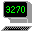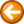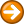DDE in an Excel MacroThe following is a sample Excel VB macro using DDE to pass data from Excel to TN3270 Plus.

Sub DDESample()

' Initiate a DDE connection to TN3270 Plus session "Mainframe"

channel = DDEInitiate(App:="tn3270", Topic:="Mainframe")

' Put TN3270 Plus screen locations for the poke operations in Excel cells A1 and A2

ActiveCell.Range("A1") = 81"

ActiveCell.Range("A2") = "161"

' Put the data to poke into the TN3270 Plus fields into Excel cells A3 and A4.

ActiveCell.Range("A3") = "555"

ActiveCell.Range("A4").NumberFormat = "@"       ' Format this cell as a string.

ActiveCell.Range("A4") = "0005093075236054321"

' Save the updates to cells A1 - A4

ActiveWorkbook.Save

' Move the cursor to the first field

Set rangeToPoke = Worksheets("Sheet1").Range("A1")

Application.DDEPoke channel, "Cursor", rangeToPoke

' Write data to the first TN3270 Plus field

Set rangeToPoke = Worksheets("Sheet1").Range("A3")

DDEPoke channel, "Keystroke", rangeToPoke

' Move the cursor to the second field

Set rangeToPoke = Worksheets("Sheet1").Range("A2")

Application.DDEPoke channel, "Cursor", rangeToPoke

' Write data to the second TN3270 Plus field

Set rangeToPoke = Worksheets("Sheet1").Range("A4")

DDEPoke channel, "Keystroke", rangeToPoke

' Terminate the DDE session

Application.DDETerminate channel

End Sub